# RBSE Solutions For Class 10 Science Chapter 10: Electric Current | Textbook Important Questions & Answers

RBSE Class 10 Science Chapter 10 Electric Current Solutions is the best resource for the students to get a complete overview of the concepts taught for the academic year from Chapter 10 of Class 10 Science textbook. Electric current is the rate of flow of current in a conducting wire from high potential to low potential. Chapter 10 of RBSE Class 10 Science, deals in-depth with concepts like Electric current, Ohm’s Law, Resistance, Combination of Resistance and so on. Understanding these concepts and topics requires diligent practice, commitment and hard work. To help with this preparation, we have given here the list of best questions and solutions that can help the students to prepare most efficiently for the board exams. RBSE Class 10 Solutions of Chapter 10 Science, Electric Current help the students to master the concepts and learn the subject thoroughly.

We have listed here the chapter-wise questions with solutions from the Rajasthan Board Class 10 Chapter 10 Science Solutions of RBSE. These questions cover all the key highlights from the chapter and also consist of solutions to the questions asked at the end of the chapter in the textbook.

## Rajasthan Board Class 10 Science Chapter 10- BYJU’S Important Questions & Answers

### RBSE Class 10 Science Chapter 10 Objective Questions-Important Questions and Solutions

1. If 2 A current flows in a conductor connected with a 5 V battery, find the resistance of the conductor.

(a) 3 Ω

(b) 2.5 Ω

(c) 10 Ω

(d) 2 Ω

2. Resistivity depends on which of the following?

(a) Length of conductor

(b) Transverse section of conductor

(c) Material of conductor

(d) None of these

3. Volt is the unit of ______

(a) Current

(b) Potential difference

(c) Charge

(d) Work

4. Three conducting wires of 1 Ω, 2 Ω and 3 Ω are connected in series in an electrical circuit. What will be the equivalent resistance?

(a) > 1 Ohm

(b)> 3 Ohm

(c) < 1 Ohm

(d) < 3 Ohm

5. The frequency of Alternating Current in India is_____

(a) 55 Hz

(b) 50 Hz

(c) 60 Hz

(d) 45 Hz

6. Resistances of different values are connected in parallel and connected with an electrical source. In each of the resistor______

(a) Current and potential difference will be different

(b) Current and potential difference will be the same

(c) Current will be different but potential difference will be same

(d) Current will be same but potential difference will be different

Answer: (c) Current will be different but potential difference will be same

7. 2 coulomb charge passes through an electrical circuit in 0.5 second. Find the value of the electric current in ampere.

(a) 1 ampere

(b) 4 ampere

(c) 1.5 ampere

(d) 10 ampere

8. Which of the devices does not depend on thermal effect of current?

(a) Heater

(b) Electric Current

(c) Toaster

(d) Refrigerator

9. Why are several appliances connected in parallel to each other in household circuits?

(a) The appliances operate at same voltage

(b) The appliances can be operated independent of each other

(c) Even if a component of a electric circuit fails other can work efficiently

(d) All of the above

Answer: (d) All of the above

10. The resistivity of a wire_________

(a) varies with its mass

(b) varies with its length

(c) varies with its cross section

(d) is independent of length, cross section and mass of wire

Answer: (d) is independent of length, cross section and mass of wire

### RBSE Class 10 Science Chapter 10 Very Short Answer Questions-Important Questions and Solutions

1. What is the unit of specific resistance or resistivity?

Answer: The unit of specific resistance or resistivity is Ohm meter.

2. Define the electric current.

Answer: The flow of electric charge in a conducting wire from high potential to low potential is called electric current.

3. What is the potential difference?

Answer: An electric potential is also called the electric field potential or the electrostatic potential. It is the amount of the electric potential energy, which a unitary point electric charge will have if located at any point in space. Potential difference is the difference in two points in a circuit. The potential difference between two points of an electric circuit is defined by work.

4. What is 1 ohm resistance?

Answer: The resistance of the conductor that produces a potential difference of 1 V between its two ends, when 1 A of electric current is passed through it is 1 Ω.

5. How does resistance depend upon cross section area?

Answer: As the cross sectional area of the wire increases, the resistance of the wire decreases. Resistance is inversely proportional to the area of the cross section.

6. Define resistivity?

Answer: The resistance of wire that has unit length and unit cross sectional area is called specific resistance or resistivity of the material.

7. What do you mean by electric power?

Answer: Electric power is the work done by the electric current in unit time.

8. 100W – 220V is written on an electric bulb. What does it mean?

Answer: 100 W is the power of a bulb. At the same time, the bulb should have 220 V maximum potential difference to function properly.

9. How is an electrical combination made at home?

Answer: The parallel combination type of resistors are used at home.

### RBSE Class 10 Science Chapter 10 Short Answer Questions-Important Questions and Solutions

10. What is the difference between series combination and parallel combination of resistances?

Answer: Given here is the table listing out the points showing the differences between a series combination and a parallel combination of resistances:

 Series Combination Parallel Combination In this second end of the first wire is connected with the first end of the second wire, and the second end of the second wire with the first end of the third wire and so on. All the resistances are connected in such a way that one end of each of them is connected at one point, C and the other end of each of them is connected to another point, D. Same current flows from all resistances Current of each resistance is different Potential difference across their ends is different Potential difference across each resistance is the same

11. What is the electric power? Write the necessary formula for it.

Answer: Electric power is the work done per second when current flows through an electric circuit.

Electric Power (P) is calculated by dividing the Work done (W) with Total Time (t). Hence, P= W/t . Also the energy given to a pure resistor is marked as VI t. Therefore, the power given to the resistor is calculated with

P= W / t = (VI t) / t [Given that V = IR]

P =VI

P = IR × I

P= I2R

Meanwhile, the unit of power is joules/ second, also referred to as watt.

12. Two resistances are of the same material and having the same length. If the ratio of their cross sectional area is 2:11, then what will be the ratio of their differences?

Answer: Given that R = K × 1/ A and

R and r are resistance of two wires with A2 as the area of the cross-section

Then, R/ r = 1/ A1 + 1/A2

Hence, A2 / A1 = 11/2.

13. What is the difference between Alternating Current Generator and Direct Current Generator?

Answer: Given below are the differences between AC and DC Generator:

 Alternating Current Generator Direct Current Generator Two slip rings SIngle slip ring commutator Current flows in both direction Current flow only in one direction

14. Write the right hand rule.

Answer: When a right handed screw is rotated circularly, and the point of the screw moves in the direction of the electric current then the direction of the rotation of the screw will show in the direction of the magnetic field. According to this law if conductor wire is held by the right hand such that the thumb is in the direction of the flow of the current then the curved fingers will represent the direction of the magnetic field. This is the right hand law.

15. Find the number of joules in 1 kilowatt hour

Answer: Here, 1 kilowatt= 1000 Watt= 103 Watt

and t=1 hour= 60 x 60.

Electrical energy is the multiplication of electrical energy into time.

Electrical Energy = P x t

1 Kilowatt/ Hour = 1000 x 60 x 60 watt x second

= 103 x 60 x 60

= 105 x 36 x (joule/second) x second

= 36 x 105 joule.

16. Write Joule’s law of heating.

Answer: Heat generated in the resistance wire is:

(a) Proportional to the square of the current flowing in the wire

H ∝ I2

(b) Proportional to the resistance

H ∝ R

(c) Proportional to the time t of flow of current

H ∝ t

All three laws are known as the joule’s law of heating.

17. Give a circuit diagram of experimental verification of Ohm’s law.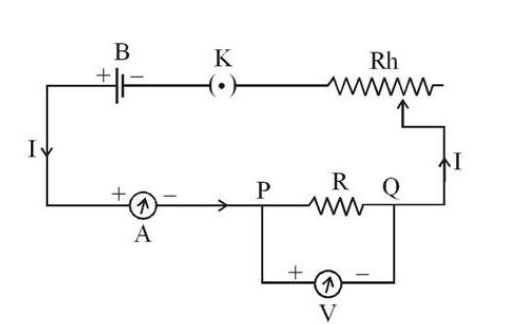### RBSE Class 10 Science Chapter 10 Essay Questions-Important Questions and Solutions

18. Explain construction and the working of alternating current generators. Draw the necessary diagram.

Answer: A device that converts mechanical energy to alternating current energy, is the AC generator. The Alternating current generator has four major components. They are (a) Field magnet, (b) Armature or coil (c) Slip Rings and (d) Brushes.

(a) Field magnet- A powerful magnet in the horseshoe shape is shown as NS

(b) Armature or coil-Copper coil ABCD is wound around a structure of cast iron

(c) Slip Rings- End A and D of the armature coil are connected separately to the slip rings S1 and S2, which are insulated from each other. The slip rings rotate along the coil

(d) Brushes- Made of graphite or carbon, one end of the brush is in contact with the slip rings and the other end is connected to the outer circuit. They rotate the armature coil. For further details on the AC Generator and its working principles, check out the link.

19. Derive the necessary formula for equivalent resistance of a series combination of resistances giving a proper circuit diagram.

Answer: The series combination of three conducting wires, AB, BC and CD are given in this figure below: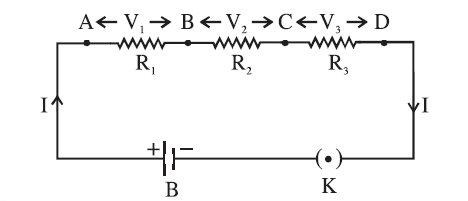Given that R1, R2 and R3 are their resistances and In a series combination, same current I flows from all the resistances. Meanwhile, the developed potential difference across the across the ends of resistances are V1,V2 and V3. Meanwhile, as per the Ohm’s law, the

Potential difference across the wire AB

R1 V1=IR1

Potential difference across the wire BC

R2 V2=IR2

Potential difference across the wire CD

R3 V3=IR3

Now, if V volt is the potential difference across the battery then,

V= V1 + V2 + V3

Hence, V= IR1 + IR2 +IR3

Therefore, V= I(R1 + R2 +R3)

Since, R is the effective resistance of the series combination of R1, R2 and R3, the potential difference across the equivalent resistance is V=IR.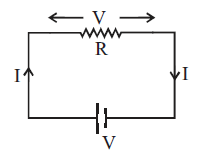Replacing this value in the equation V= IR1 + IR2 +IR3 , we get

IR= IR1 + IR2 +IR3

Or R=R1 + R2 +R3

Thus, we can say that the total resistance of a series combination of a number of resistances is equal to the sum of resistances of that combination.

20. Derive the necessary formula for equivalent resistance of a parallel combination of resistances giving a proper circuit diagram.

Answers: The figure representing Parallel combination of resistance is given below: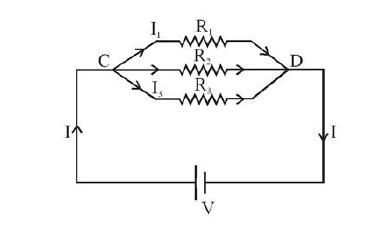R1, R2 and R3 are the resistances in a parallel combination. In Parallel connection, even if the potential difference across all the resistances are the same, the current will be different. So the current flowing through R1, R2 and R3 are represented by I1, I2 and I3, respectively.

Now, according to the Ohm’s Law,

Current in R1 ⇨ I1 = V/R1

Current in R2 ⇨ I2 = V/R2 and

Current in R3 ⇨ I3 = V/R3

Therefore, I= I1+ I2 + I3

That is I = V/R1 + V/R2 + V/R3

Or I = V [ 1/R1 + 1/R2 + 1/R3]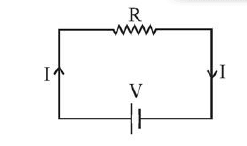Hence, if the equivalent resistance of R1, R2 and R3 in parallel combination is denoted by R, then the current flowing through the equivalent circuit is I = V/R. So, placing I=V/R , the equation would be

V/ R = V [1/R1 + 1/R2 + 1/R3]

Or 1/R = [1/R1 + 1/R2 + 1/R3]

Thus, the inverse of the equivalent resistance of a parallel combination is equal to the sum of the inverse of each of the resistance in parallel combination. Meanwhile, if there are two resistances say R1 and R2 , then

1/R = [1/R1 + 1/R2] or

1/R = (R1 + R2) / R1R2

Thus, R = R1R2/ (R1 + R2)

### RBSE Class 10 Science Chapter 10 Numerical Questions-Important Questions and Solutions

21. Find the highest and the lowest resistance from combination resistances of 1Ω, 2Ω and 3Ω.

Answers: The highest resistance is obtained using the Series combination formula, while the lowest resistance can be obtained from Parallel combination formula.

In a series combination; the equivalent resistance, R = R1 + R2 + R3. Replacing the resistances with values, R = 1Ω + 2Ω + 3Ω = 6Ω. Hence, the highest resistance is 6 Ohm.

Meanwhile, equivalent resistance of Parallel combination is

1/ R= 1/R1 + 1/R2 + 1/R3

1/ R = 1/1 + ½ + ⅓

=(6+ 3+2) / 6

= 11/6 Ω

1/ R = 11/6Ω

Hence, R= 6/ 11Ω. From this, you can see that the lowest resistance is 6/ 11Ω. Ohm.

22. What will be the resistance of a conductor wire when the potential difference across its terminals is 2.5 volt when 10 milliampere current flows through it.

Answer: Given that I = 10 milliampere = 0.01A and V= 2.5 volt . make use of Ohm’s law

That is R = V/I

Therefore, R= 2.5 V /0.01A = 250 Ω.

Hence, the resistance of a conductor wire is 250 Ω.

23. Find the equivalent resistance between A and B in following circuits: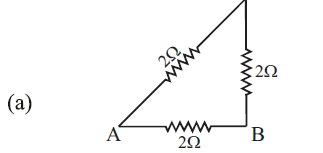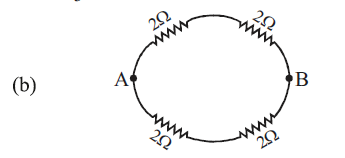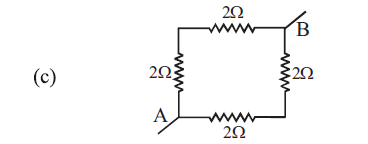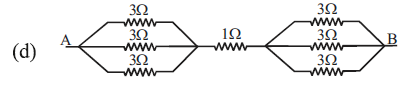Answer: (a) Here, R1 , R2 and R3 = 2Ω

Given that two resistors are connected in series and third resistor is in parallel to this group, the equivalent resistance of two resistors in series is given as

R12 = R1+ R2 = 2Ω + 2Ω = 4Ω

Meanwhile, the equivalent resistance in parallel is calculated as

1/ R = 1/ R12 + 1/ R3

= ¼ + ½

= (1+2) /4 = ¾

Thus, if 1/ R = ¾ , then

R= 4/3 Ω

(b) Given that two groups of two resistors are each in series combination and are attached in parallel combination, the equivalent resistance in series combination is given below:

R12 = R1 + R2= 2Ω + 2Ω = 4Ω

R34 = R3 + R4 = 2Ω + 2Ω = 4Ω

Thus, the equivalent resistance in parallel combination can be calculated with the formula:

1/R = 1/R12 + 1/ R34

= ¼ + ¼ = 2/4 = ½

Hence, 1/R = ½

Thus. R will be equal to 2Ω.

(c) Given that two groups of two resistors are each in series combination and are attached in parallel combination, the equivalent resistance in series combination is given below:

R12 = R1 + R2= 2Ω + 2Ω = 4Ω

R34 = R3 + R4 = 2Ω + 2Ω = 4Ω

Thus, the equivalent resistance in parallel combination can be calculated with the formula:

1/R = 1/R12 + 1/ R34

= ¼ + ¼ = 2/4 = ½

Hence, 1/R = ½

Thus. R will be equal to 2Ω.

(d) Given here are three groups of resistors in series, while two groups have three resistors in parallel combination. Then, see the equivalent resistance in group of resistors in LHS (Parallel Combination), calculated by

1/ R123 = 1R1 + 1/R2 + 1/R3

= ⅓ + ⅓ +⅓

= (1+1+1) / 3 = 3/3 = 1Ω.

Meanwhile, the equivalent resistance in a group of resistors in RHS (Parallel combination) is the same as in LHS. Thus, R = 1Ω.

Hence, the equivalent resistance for overall series combination will be = 1+1+1 = 3Ω.

24. An electric rod of 1500 watt is used for 3 hours daily to heat water. If the rate of consuming 1 electrical unit of energy is Rs 5.00, how much will be the value of consumed electrical energy in 30 days.

Answer: Find here that Power of electric rod is 1500 W = 1.5 kW

Time used per day = 3 hours and

Number of days = 30 days.

Then, calculate the power consumption in 30 days= power x t x no. of days

That is 1.5 kW x 3h x 30 = 135 kWh = 135 unit,

Therefore, if 1 unit costs Rs 5.00, then the total cost = 135 x 5 = Rs. 675

### RBSE Class 10 Science Chapter 10 Additional Questions-Important Questions and Solutions

25. Define an ammeter. How is it connected in a circuit?

Answer: An ammeter is a measuring instrument that is used to measure the potential difference between two points in an electric circuit. In a circuit, the ammeter is connected in series.

26. If the combined resistance in the given circuit is 5 Ω, then find the value of R.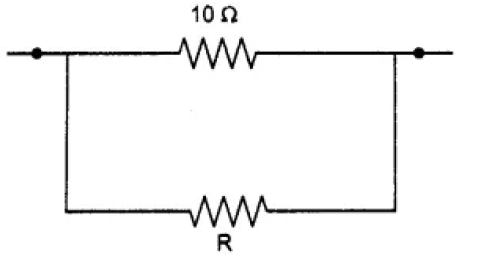Answer: Given here is a parallel connection, hence 1/ R = 1/ R1 + 1/ R2,. Replacing the equation with the numerical values, you get ⅕ = 1/10 + 1/ R2.

Therefore, 1/ R2 = ⅕ -1/10 .

Hence, R2 = 10Ω

27. What is a magnetic field? Which are the factors that the magnetic field due to current carrying conductor depend on?

Answer: The magnetic field is the area around the magnet, where the force of attraction or repulsion produced by the magnet is detected. Meanwhile, the magnitude of the magnetic field at the given point increases as the current through the wire increases. At the same time, it decreases with the increase in distance from the wire.

28. Point out the differences between resistance and resistivity.

Answer: Resistance is the property of the conductor to resist the flow of charge through it, while resistivity is the resistance per unit length of a unit cross section of the material. The unit for resistance is Ohm, while for resistivity it is Ohm meter. Finally, resistance is proportional to the length, cross sectional area of the wire and material medium that depends on the temperature. Alternatively, the resistivity is constant for a conductor only at a given temperature. It varies with the temperature. Along with the rise in temperature of metals and alloys, the resistivity also increases.

29. Define purely resistive electric circuit.

Answer: If the resistor of a circuit is connected to the battery so that the source energy gets dissipated completely in the form of heat, then it is called a purely resistive electric circuit.

30. What is magnetic flux?

Answer: The number of magnetic lines of force passing through a surface, kept in the magnetic field, is called the magnetic flux, associated with that surface.

During the exams, the RBSE solutions Class 10 will help the students to solve all their doubts. Preparing these solutions will boost the students’ exam preparation and help them ace the board exam with flying colors.

Find here at BYJU’S other resources such as RBSE Textbooks, syllabus and question papers to study well for the exams.